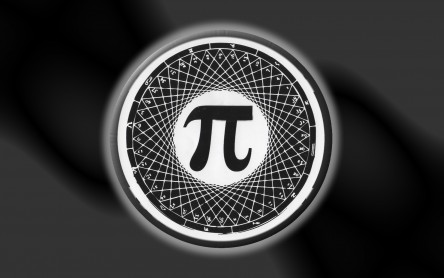# (Repost) Circles And Squares – Think Outside The Box With The Number Pi (mpHop.com)Pizza – The REAL American 3.14! Spell it out..”Pi”-zza. Image provided by Â  Â  Â  Â  Â  Â  Â  Â  www.pie-zans.com

(Editors Note – Thursday March 14, 2013)
Originally Published on: Oct 16, 2011 @ 5:15

### Pi Day

Pi Day is an annual celebration commemorating the mathematical constant Ï€ (pi). Pi Day is observed on March 14 (or 3/14 in month/day date format), since 3, 1 and 4 are the three most significant digits of Ï€ in the decimal form. In 2009, the United States House of Representatives supported the designation of Pi Day.

Pi Approximation Day is observed on July 22 (or 22/7 in day/month date format), since the fraction 22â„7 is a common approximation of Ï€.

Source: en.wikipedia.org/wiki/Pi_Day

(End Note)# Pi

Ï€ (sometimes written pi) is a mathematical constant that is the ratio of any circle’s circumference to its diameter. Ï€ is approximately equal to 3.14 in the usual decimal notation. Many formulae in mathematics, science, and engineering involve Ï€, which makes it one of the most important mathematical constants. For instance, the area of a circle is equal to Ï€ times the square of the radius of the circle.

Ï€ is an irrational number, which means that its value cannot be expressed exactly as a fraction having integers in both the numerator and denominator. Consequently, its decimal representation never ends or repeats. Ï€ is also a transcendental number, which implies, among other things, that no finite sequence of algebraic operations on integers (powers, roots, sums, etc.) can render its value; proving this fact was a significant result of 19th century German mathematics.

Throughout the history of mathematics, there has been much effort to determine Ï€ more accurately and to understand its nature; fascination with the number has even carried over into non-mathematical culture. Probably because of the simplicity of its definition, the concept of Ï€ has become entrenched in popular culture to a degree far greater than for almost any other mathematical concept. It is, perhaps, the most common ground between mathematicians and non-mathematicians. Reports on the latest, most-precise calculation of Ï€ are common news items. The current record for the decimal expansion of Ï€, if verified, stands at 5 trillion digits.

Source: en.wikipedia.org/wiki/Pi

Now how deep is The Game really?
You do the math.The circumference of a circle is slightly more than three times as long as its diameter. The exact ratio is called Ï€.

Because Ï€ is a transcendental number, squaring the circle is not possible in a finite number of steps using the classical tools of compass and straightedge

Become A Part Of The Solution!

Ï€mpHop.com Lifestyle Magazine